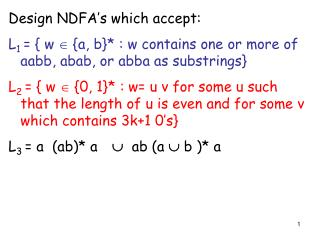Download PresentationDesign NDFA’s which accept:

# Design NDFA’s which accept: - PowerPoint PPT PresentationDownload Presentation## Design NDFA’s which accept:

- - - - - - - - - - - - - - - - - - - - - - - - - - - E N D - - - - - - - - - - - - - - - - - - - - - - - - - - -
##### Presentation Transcript

1. Design NDFA’s which accept: L1 = { w  {a, b}* : w contains one or more of aabb, abab, or abba as substrings} L2 = { w  {0, 1}* : w= u v for some u such that the length of u is even and for some v which contains 3k+1 0’s} L3 = a (ab)* a  ab (a  b )* a

2. CSC 320 Lecture 9: Converting NDFA’s to DFA’s

3. Two machines M1 and M2 are equivalent if L(M1) = L(M2). Theorem: For any NDFA, there exists an equivalent DFA. Proof: By construction. Proofs by construction are nice because they don’t just tell us that an object exists- they also give us an algorithm for constructing the object.

4. E(q) = { p: p is reachable from q by following only transitions on ε}. Note: q is always in E(q). For a set S of states, E(S) = UqS E(q) Transition function for new DFA: δ(P, σ) = E(Q) where Q= {q: for some p in P, (p, σ, q) is in Δ}

5. Convert this NDFA to a DFA:

6. Start state is {s, t, x, y} = states reachable from s by traversing 0 or more e-transitions.

7. Then follow zero or more e-transitions from {s, u, v}, The next state is {s, t, u, v, x, y}.

8. Read b from {s, t, x, y} and the next state can be {x, y}.

9. Then follow zero or more e-transitions from {x, y}, The next state is {x, y}.

10. The number of possible states could be exponential. But on assignments and exams, only a small subset of them will ever be pertinent. Only work out transitions for pertinent states.

11. Convert this NDFA to a DFA:

12. Which states should be final states? Original machine: only final state was s.

13. Which states should be final states? Answer: new states whose subsets contain a state which was a final state originally.